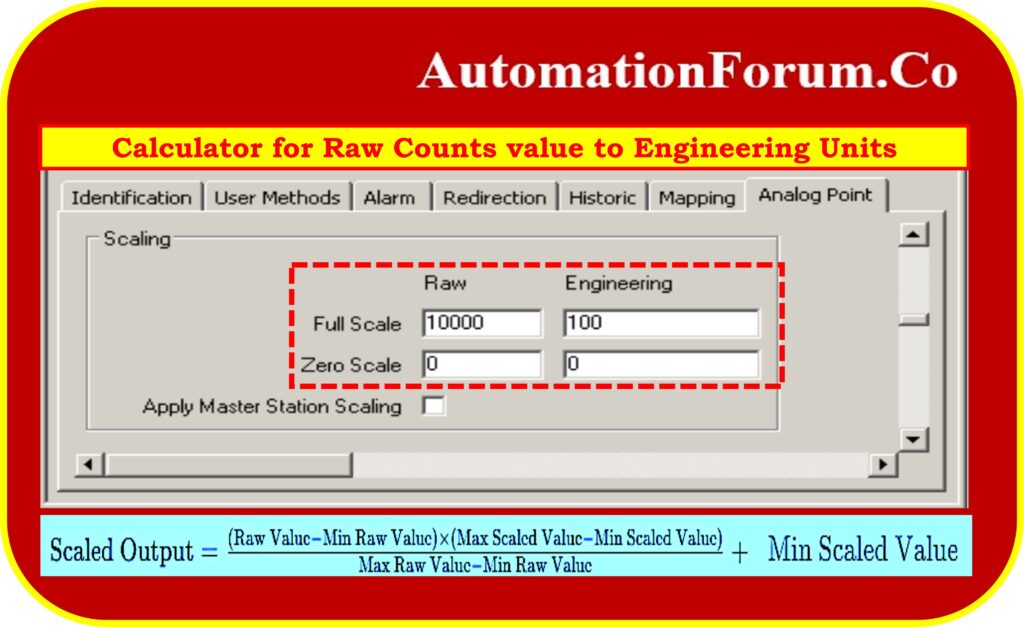# Calculator/Instrumentationtool for Raw Counts value to Engineering UnitsA calculator for raw counts to engineering units conversion is a instrumentation tool used to convert raw data obtained from sensors or measuring devices into meaningful engineering units. This conversion is crucial as the raw counts are typically in a digital format and do not directly represent physical quantities. The calculator employs calibration parameters like scale factors and offsets to perform this conversion accurately.

Within a PLC system, we need to convert the raw counts from the sensors to engineering units.

## The main uses of such a calculator include:

• Many sensors provide raw readings in specific ranges that may not be directly usable in their raw form.
• The calculator allows engineers and technicians to scale these readings to meaningful units such as temperature, pressure, distance, or any other physical quantity, making the data more comprehensible and useful for analysis.
• In industrial automation, sensors continuously monitor process parameters. Converting raw counts to engineering units allows for precise control and optimization of the process.
• The calculator is instrumental in calibrating sensors by determining the relationship between raw counts and actual physical quantities.
• Researchers and analysts use the calculator to transform raw data into engineering units for meaningful analysis and interpretation.
• Engineering units are often used in control systems to trigger specific actions or set control setpoints based on sensor readings.
• Many sensors provide data in raw counts, which need to be converted into engineering units such as temperature, pressure, distance, etc., to make the data meaningful and usable.
• Different sensors may output data in different units or ranges. Using the calculator to scale data ensures that different sensor readings can be compared and analyzed on a common scale.

## Formula for calculation

To calculate the scaled output from a raw value count, you can use the following formula:Where:

• Raw Value: The raw value count obtained from the sensor or measuring device.
• Min Raw Value: The minimum value of the raw range.
• Max Raw Value: The maximum value of the raw range.
• Min Scaled Value: The minimum value of the desired scaled output range.
• Max Scaled Value: The maximum value of the desired scaled output range.

Using this formula, you can accurately convert raw value counts from sensors or measuring devices into corresponding scaled output values within the desired output range, making the data more meaningful and usable for analysis and control purposes.

## Example calculation

We have a sensor which gives a raw value input of the range 4000 to 21000. We need the sensor value in a output scaled range of 0 to 1650. Find a formula that will allow calculating the scaled value from the raw one. I’ll make up an example: I get a raw reading of 13556 from the sensor. How can we scale that value into an output value?

You can use the following formula:

Scaled Output=

{(Raw Value−Min Raw Value)×(Max Scaled Value−Min Scaled value)}/(Max Raw Value−Min Raw Value)+Min Scaled Value

Given parameters:

• Min Raw Value = 4000
• Max Raw Value = 21000
• Min Scaled Value = 0
• Max Scaled Value = 1650

Now, plug the values into the formula:

Scaled Output = (13556−4000)×(1650−0)/(21000−4000)+0

Scaled Output = 9556×1650/17000

Scaled Output = 927.494

Therefore, a raw reading of 13556 from the sensor would correspond to a scaled output value of approximately 927.494 in the range of 0 to 1650.

This formula allows you to accurately convert raw sensor readings into corresponding scaled output values within the desired output range.

## Raw Counts to Engineering Units Calculator/Instrumentationtool

The below calculator used to determine the raw count value from the sensor to engineering units

Scroll to Top